# COLLEGE PHYSICS Chapter 18 ELECTRIC CHARGE AND ELECTRIC

• Slides: 57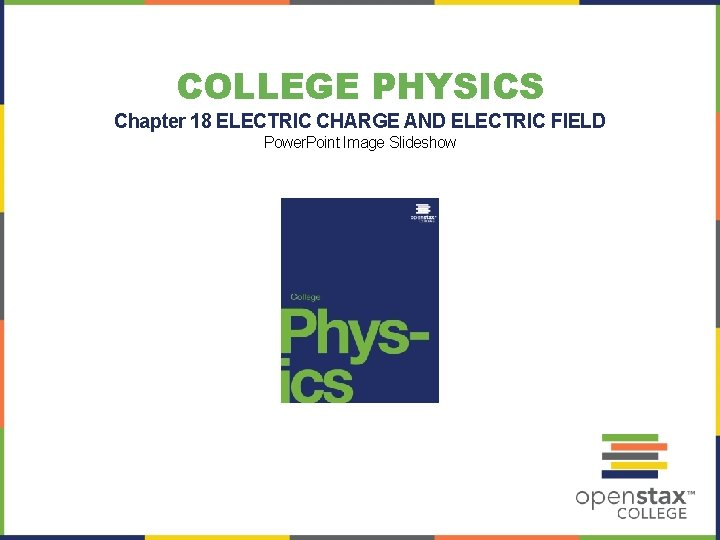COLLEGE PHYSICS Chapter 18 ELECTRIC CHARGE AND ELECTRIC FIELD Power. Point Image Slideshow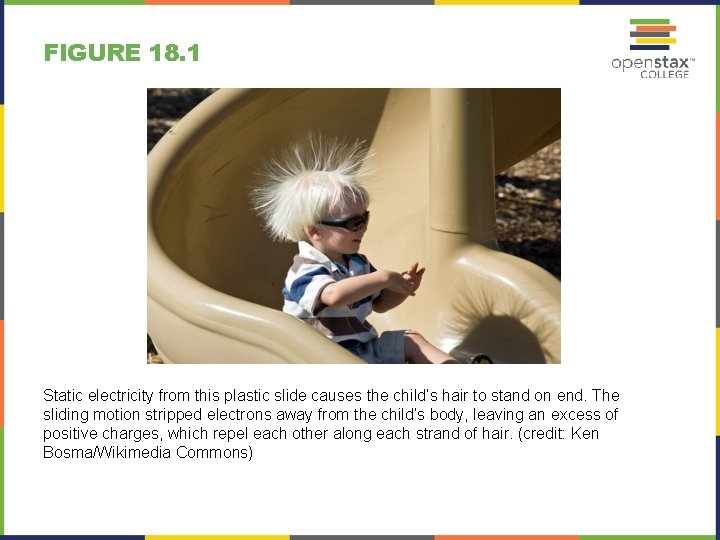FIGURE 18. 1 Static electricity from this plastic slide causes the child’s hair to stand on end. The sliding motion stripped electrons away from the child’s body, leaving an excess of positive charges, which repel each other along each strand of hair. (credit: Ken Bosma/Wikimedia Commons)FIGURE 18. 2 When Benjamin Franklin demonstrated that lightning was related to static electricity, he made a connection that is now part of the evidence that all directly experienced forces except the gravitational force are manifestations of the electromagnetic force.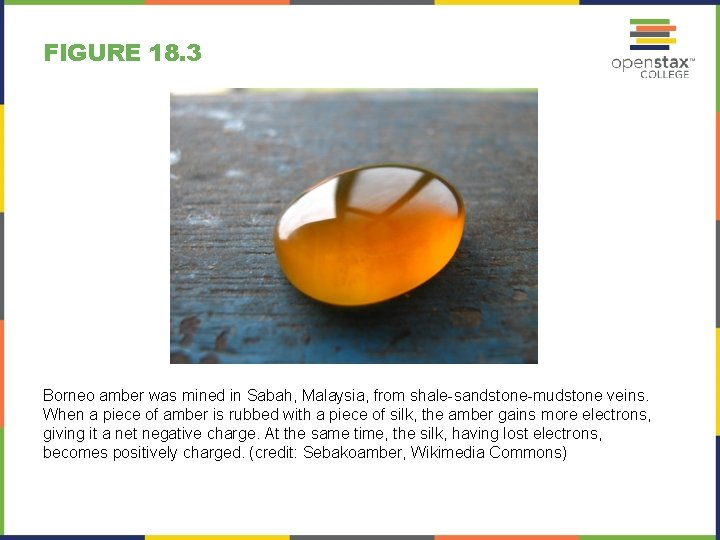FIGURE 18. 3 Borneo amber was mined in Sabah, Malaysia, from shale-sandstone-mudstone veins. When a piece of amber is rubbed with a piece of silk, the amber gains more electrons, giving it a net negative charge. At the same time, the silk, having lost electrons, becomes positively charged. (credit: Sebakoamber, Wikimedia Commons)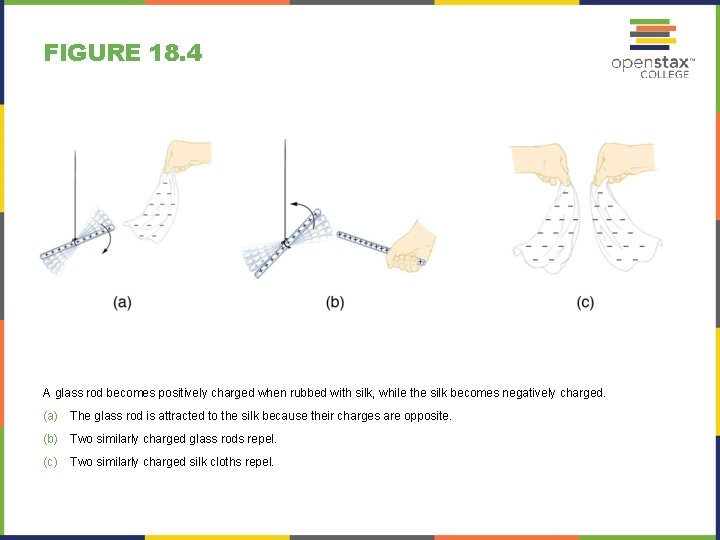FIGURE 18. 4 A glass rod becomes positively charged when rubbed with silk, while the silk becomes negatively charged. (a) The glass rod is attracted to the silk because their charges are opposite. (b) Two similarly charged glass rods repel. (c) Two similarly charged silk cloths repel.FIGURE 18. 5 This simplified (and not to scale) view of an atom is called the planetary model of the atom. Negative electrons orbit a much heavier positive nucleus, as the planets orbit the much heavier sun. There the similarity ends, because forces in the atom are electromagnetic, whereas those in the planetary system are gravitational. Normal macroscopic amounts of matter contain immense numbers of atoms and molecules and, hence, even greater numbers of individual negative and positive charges.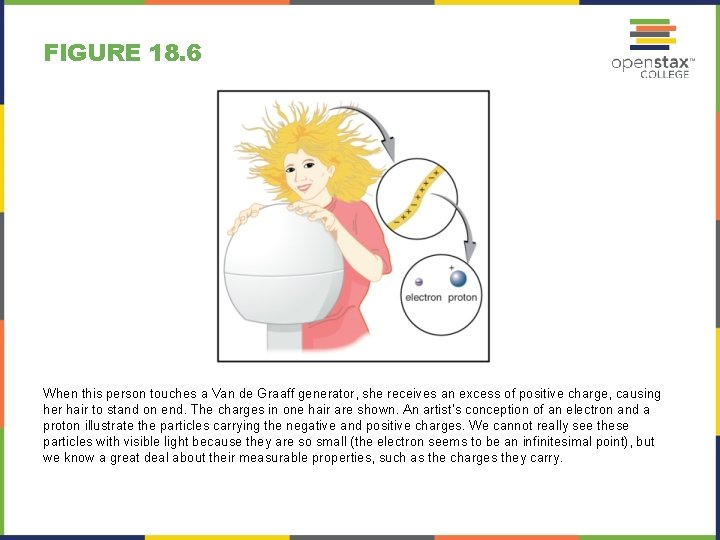FIGURE 18. 6 When this person touches a Van de Graaff generator, she receives an excess of positive charge, causing her hair to stand on end. The charges in one hair are shown. An artist’s conception of an electron and a proton illustrate the particles carrying the negative and positive charges. We cannot really see these particles with visible light because they are so small (the electron seems to be an infinitesimal point), but we know a great deal about their measurable properties, such as the charges they carry.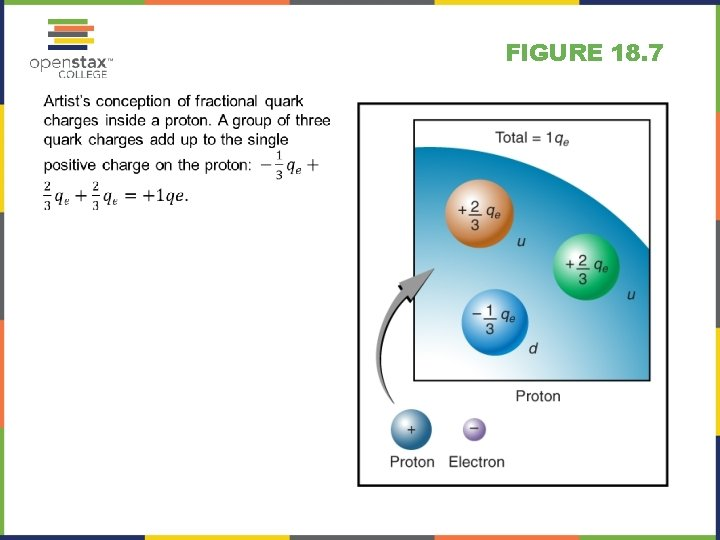FIGURE 18. 7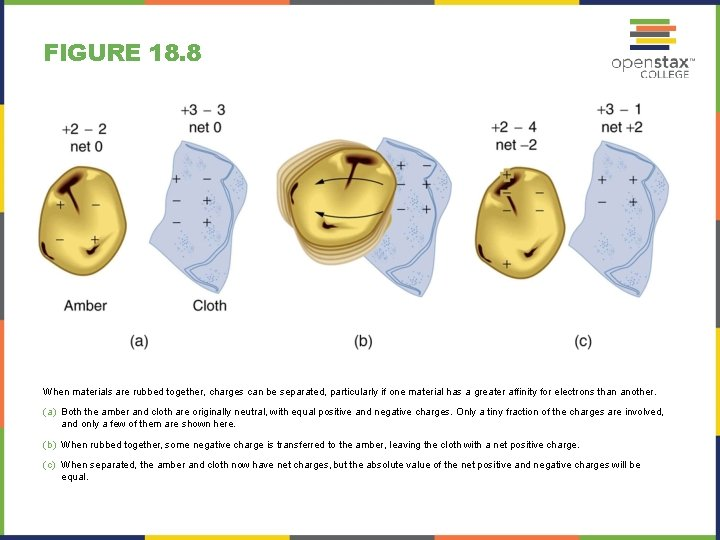FIGURE 18. 8 When materials are rubbed together, charges can be separated, particularly if one material has a greater affinity for electrons than another. (a) Both the amber and cloth are originally neutral, with equal positive and negative charges. Only a tiny fraction of the charges are involved, and only a few of them are shown here. (b) When rubbed together, some negative charge is transferred to the amber, leaving the cloth with a net positive charge. (c) When separated, the amber and cloth now have net charges, but the absolute value of the net positive and negative charges will be equal.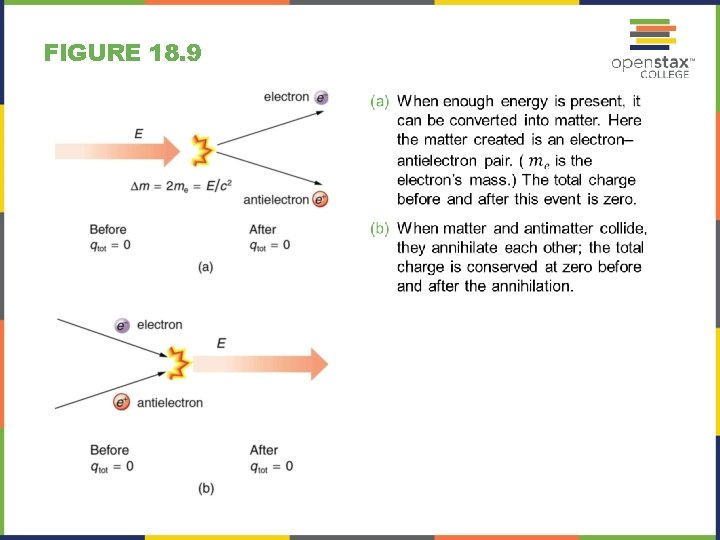FIGURE 18. 9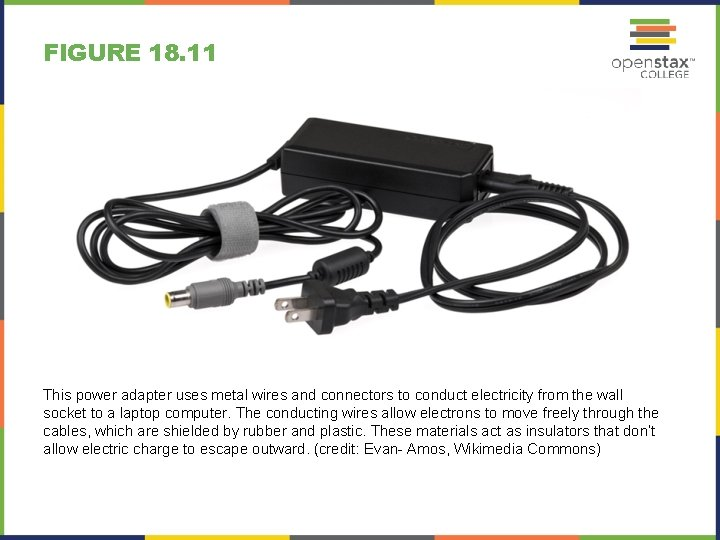FIGURE 18. 11 This power adapter uses metal wires and connectors to conduct electricity from the wall socket to a laptop computer. The conducting wires allow electrons to move freely through the cables, which are shielded by rubber and plastic. These materials act as insulators that don’t allow electric charge to escape outward. (credit: Evan- Amos, Wikimedia Commons)FIGURE 18. 12 An electroscope is a favorite instrument in physics demonstrations and student laboratories. It is typically made with gold foil leaves hung from a (conducting) metal stem and is insulated from the room air in a glass-walled container. (a) A positively charged glass rod is brought near the tip of the electroscope, attracting electrons to the top and leaving a net positive charge on the leaves. Like charges in the light flexible gold leaves repel, separating them. (b) When the rod is touched against the ball, electrons are attracted and transferred, reducing the net charge on the glass rod but leaving the electroscope positively charged. (c) The excess charges are evenly distributed in the stem and leaves of the electroscope once the glass rod is removed.FIGURE 18. 13 Charging by induction. (a) Two uncharged or neutral metal spheres are in contact with each other but insulated from the rest of the world. (b) A positively charged glass rod is brought near the sphere on the left, attracting negative charge and leaving the other sphere positively charged. (c) The spheres are separated before the rod is removed, thus separating negative and positive charge. (d) The spheres retain net charges after the inducing rod is removed—without ever having been touched by a charged object.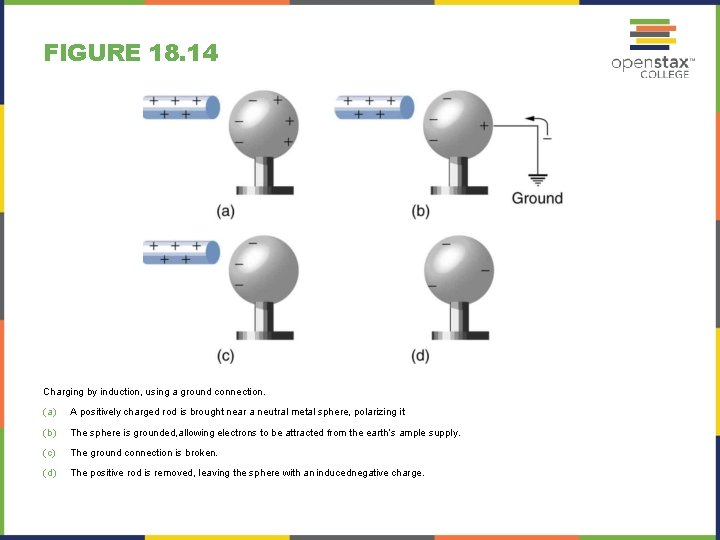FIGURE 18. 14 Charging by induction, using a ground connection. (a) A positively charged rod is brought near a neutral metal sphere, polarizing it. (b) The sphere is grounded, allowing electrons to be attracted from the earth’s ample supply. (c) The ground connection is broken. (d) The positive rod is removed, leaving the sphere with an inducednegative charge.FIGURE 18. 15 Both positive and negative objects attract a neutral object by polarizing its molecules. (a) A positive object brought near a neutral insulator polarizes its molecules. There is a slight shift in the distribution of the electrons orbiting the molecule, with unlike charges being brought nearer and like charges moved away. Since the electrostatic force decreases with distance, there is a net attraction. (b) A negative object produces the opposite polarization, but again attracts the neutral object. (c) The same effect occurs for a conductor; since the unlike charges are closer, there is a net attraction.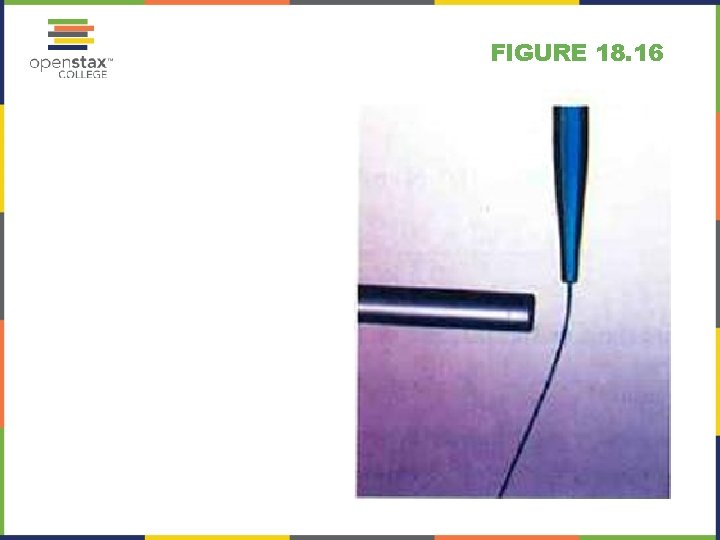FIGURE 18. 16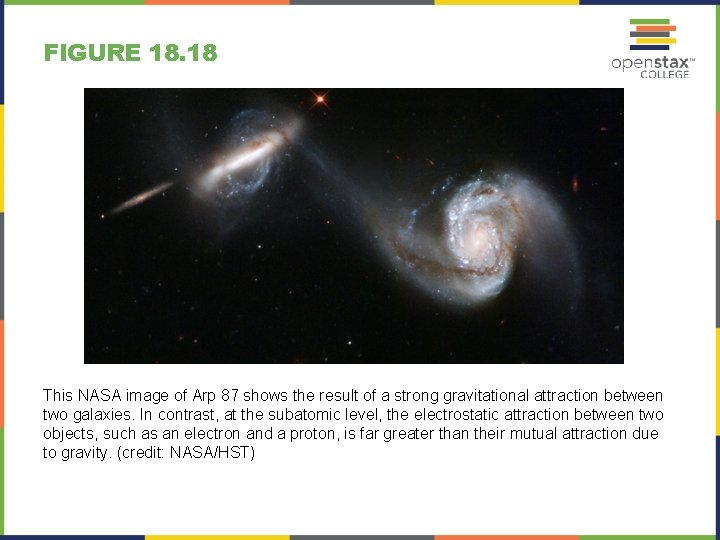FIGURE 18. 18 This NASA image of Arp 87 shows the result of a strong gravitational attraction between two galaxies. In contrast, at the subatomic level, the electrostatic attraction between two objects, such as an electron and a proton, is far greater than their mutual attraction due to gravity. (credit: NASA/HST)FIGURE 18. 19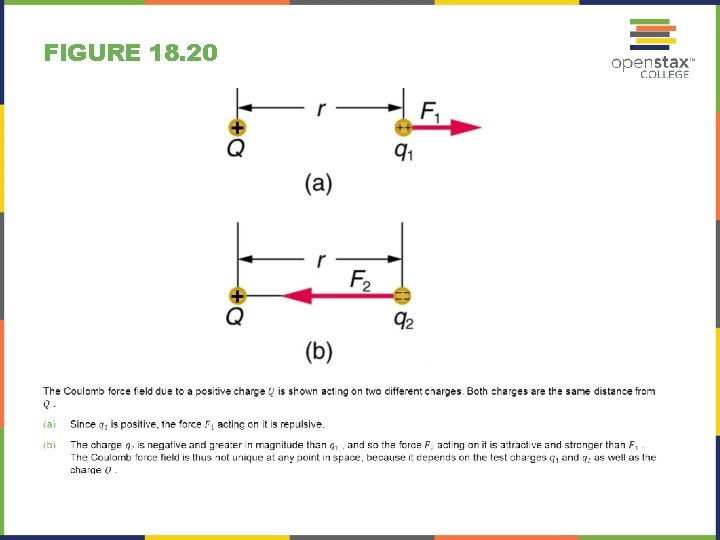FIGURE 18. 20FIGURE 18. 22 Two equivalent representations of the electric field due to a positive charge Q. (a) Arrows representing the electric field’s magnitude and direction. (b) In the standard representation, the arrows are replaced by continuous field lines having the same direction at any point as the electric field. The closeness of the lines is directly related to the strength of the electric field. A test charge placed anywhere will feel a force in the direction of the field line; this force will have a strength proportional to the density of the lines (being greater near the charge, for example).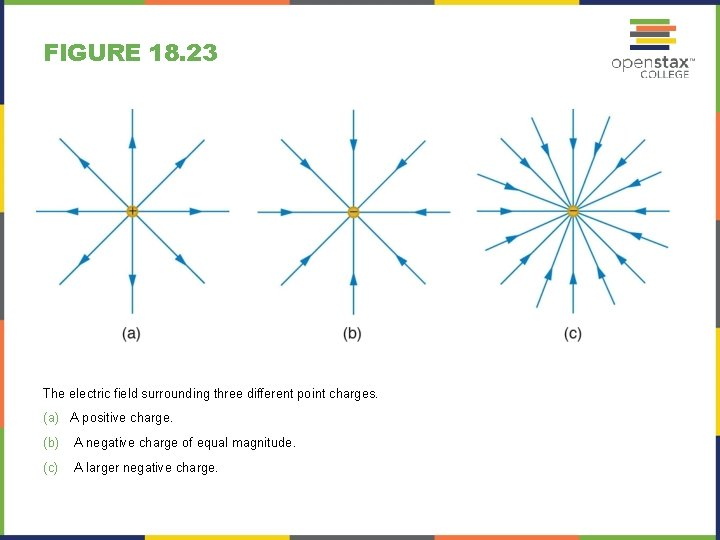FIGURE 18. 23 The electric field surrounding three different point charges. (a) A positive charge. (b) A negative charge of equal magnitude. (c) A larger negative charge.FIGURE 18. 24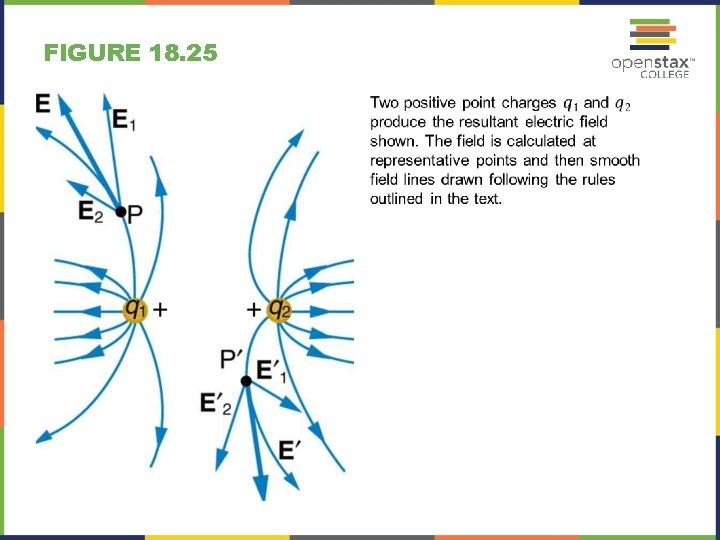FIGURE 18. 25FIGURE 18. 26 (a) Two negative charges produce the fields shown. It is very similar to the field produced by two positive charges, except that the directions are reversed. The field is clearly weaker between the charges. The individual forces on a test charge in that region are in opposite directions. (b) Two opposite charges produce the field shown, which is stronger in the region between the charges.FIGURE 18. 28 DNA is a highly charged molecule. The DNA double helix shows the two coiled strands each containing a row of nitrogenous bases, which “code” the genetic information needed by a living organism. The strands are connected by bonds between pairs of bases. While pairing combinations between certain bases are fixed (C-G and A-T), the sequence of nucleotides in the strand varies. (credit: Jerome Walker)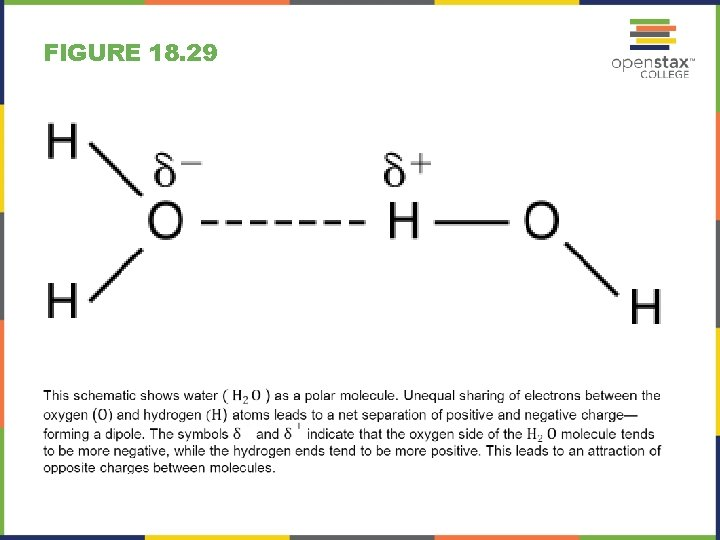FIGURE 18. 29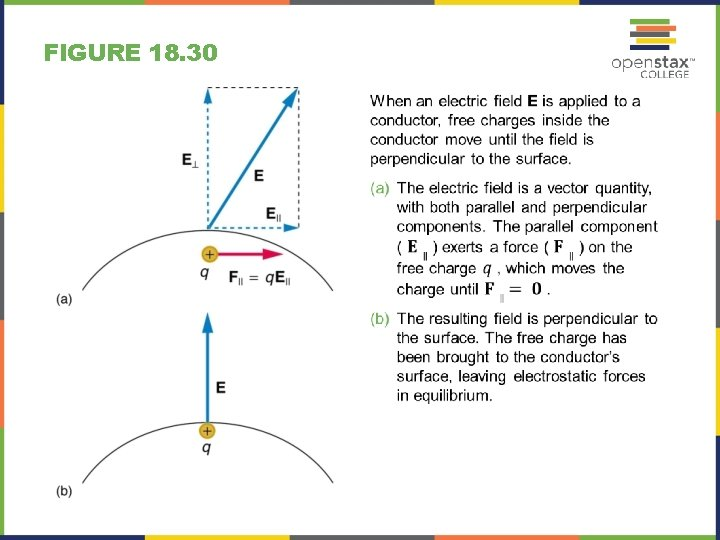FIGURE 18. 30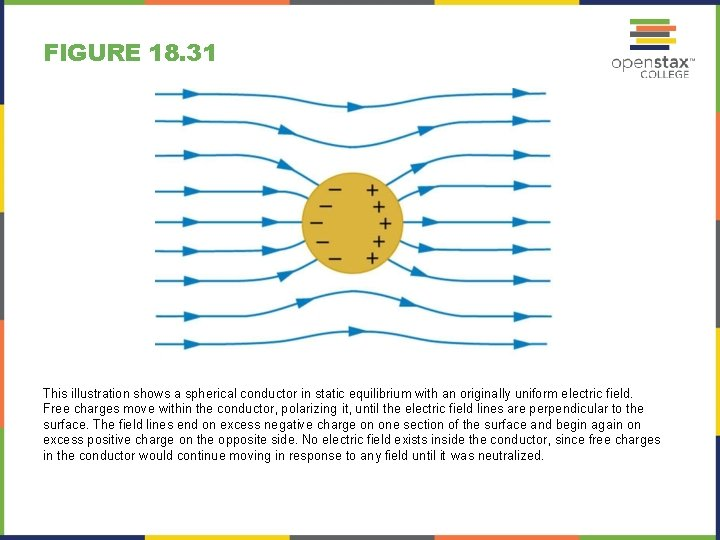FIGURE 18. 31 This illustration shows a spherical conductor in static equilibrium with an originally uniform electric field. Free charges move within the conductor, polarizing it, until the electric field lines are perpendicular to the surface. The field lines end on excess negative charge on one section of the surface and begin again on excess positive charge on the opposite side. No electric field exists inside the conductor, since free charges in the conductor would continue moving in response to any field until it was neutralized.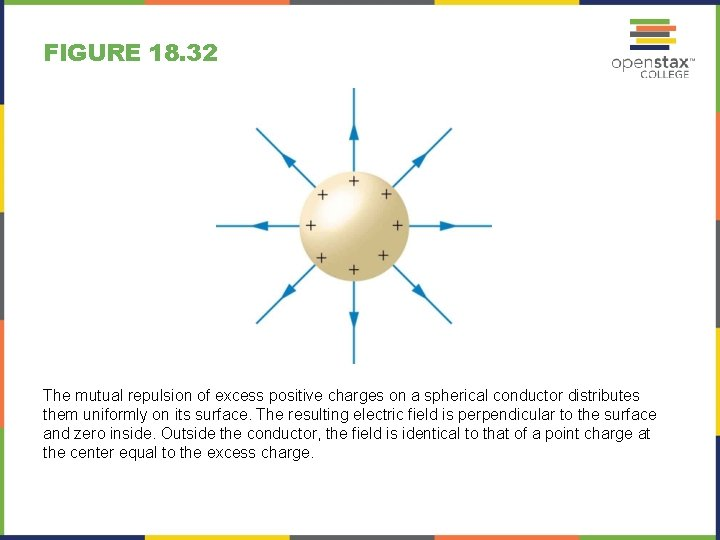FIGURE 18. 32 The mutual repulsion of excess positive charges on a spherical conductor distributes them uniformly on its surface. The resulting electric field is perpendicular to the surface and zero inside. Outside the conductor, the field is identical to that of a point charge at the center equal to the excess charge.FIGURE 18. 33 Two metal plates with equal, but opposite, excess charges. The field between them is uniform in strength and direction except near the edges. One use of such a field is to produce uniform acceleration of charges between the plates, such as in the electron gun of a TV tube.FIGURE 18. 34 Earth’s electric field. (a) Fair weather field. Earth and the ionosphere (a layer of charged particles) are both conductors. They produce a uniform electric field of about 150 N/C. (credit: D. H. Parks) (b) Storm fields. In the presence of storm clouds, the local electric fields can be larger. At very high fields, the insulating properties of the air break down and lightning can occur. (credit: Jan-Joost Verhoef)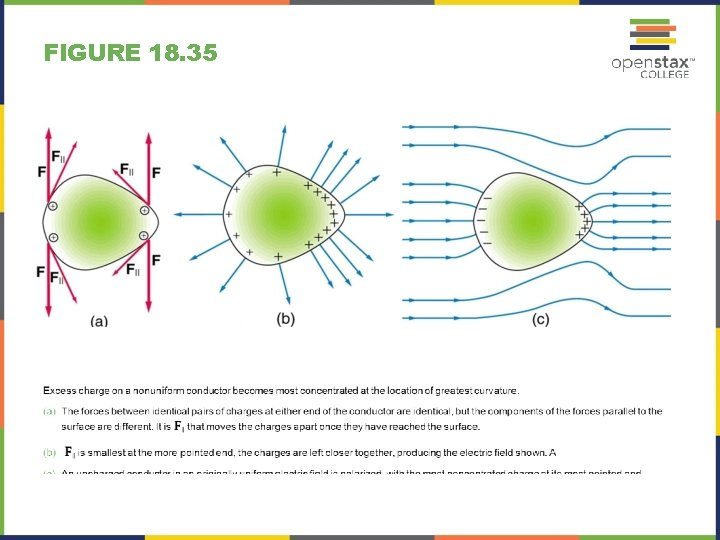FIGURE 18. 35FIGURE 18. 36 A very pointed conductor has a large charge concentration at the point. The electric field is very strong at the point and can exert a force large enough to transfer charge on or off the conductor. Lightning rods are used to prevent the buildup of large excess charges on structures and, thus, are pointed.FIGURE 18. 37 (a) A lightning rod is pointed to facilitate the transfer of charge. (credit: Romaine, Wikimedia Commons) (b) This Van de Graaff generator has a smooth surface with a large radius of curvature to prevent the transfer of charge and allow a large voltage to be generated. The mutual repulsion of like charges is evident in the person’s hair while touching the metal sphere. (credit: Jon ‘Shakata. Ga. Nai’ Davis/Wikimedia Commons).FIGURE 18. 38 Schematic of Van de Graaff generator. A battery (A) supplies excess positive charge to a pointed conductor, the points of which spray the charge onto a moving insulating belt near the bottom. The pointed conductor (B) on top in the large sphere picks up the charge. (The induced electric field at the points is so large that it removes the charge from the belt. ) This can be done because the charge does not remain inside the conducting sphere but moves to its outside surface. An ion source inside the sphere produces positive ions, which are accelerated away from the positive sphere to high velocities.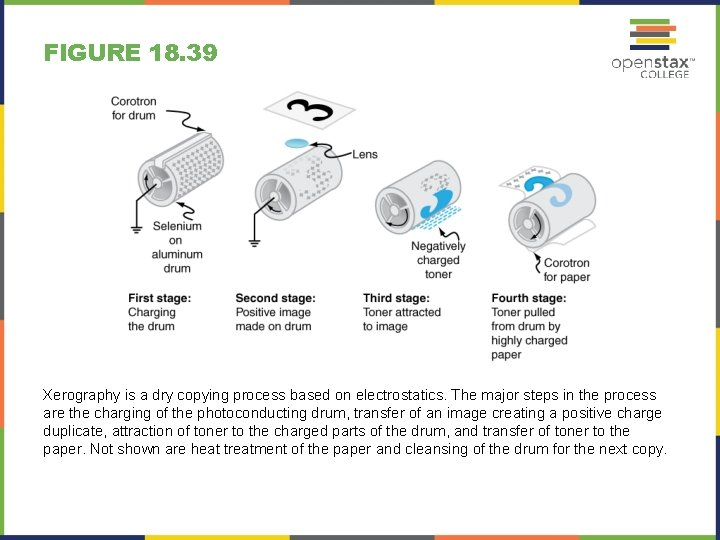FIGURE 18. 39 Xerography is a dry copying process based on electrostatics. The major steps in the process are the charging of the photoconducting drum, transfer of an image creating a positive charge duplicate, attraction of toner to the charged parts of the drum, and transfer of toner to the paper. Not shown are heat treatment of the paper and cleansing of the drum for the next copy.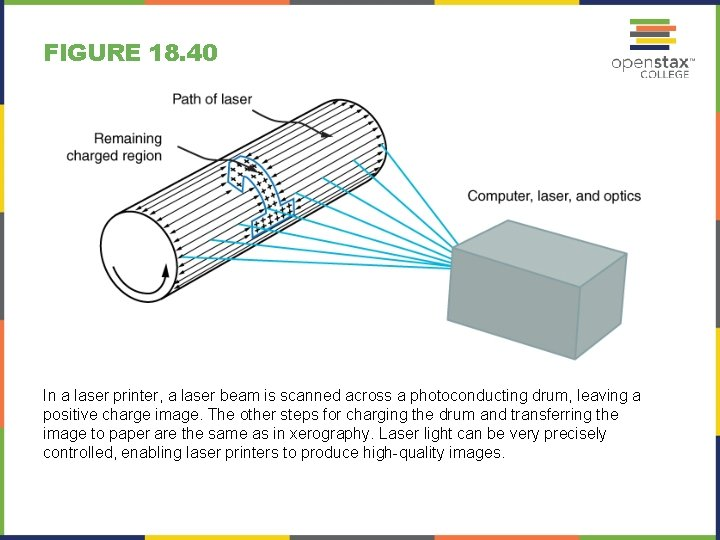FIGURE 18. 40 In a laser printer, a laser beam is scanned across a photoconducting drum, leaving a positive charge image. The other steps for charging the drum and transferring the image to paper are the same as in xerography. Laser light can be very precisely controlled, enabling laser printers to produce high-quality images.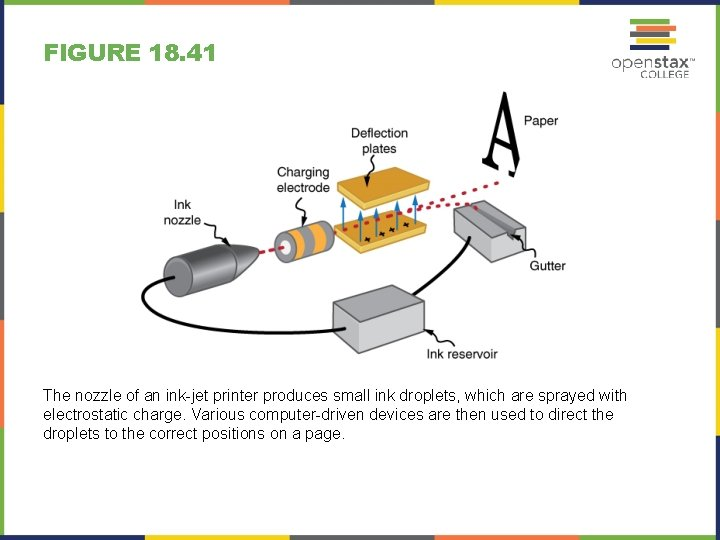FIGURE 18. 41 The nozzle of an ink-jet printer produces small ink droplets, which are sprayed with electrostatic charge. Various computer-driven devices are then used to direct the droplets to the correct positions on a page.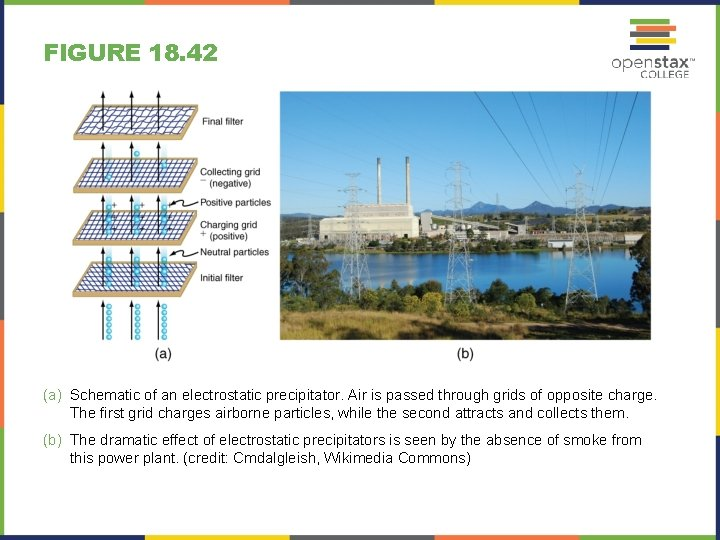FIGURE 18. 42 (a) Schematic of an electrostatic precipitator. Air is passed through grids of opposite charge. The first grid charges airborne particles, while the second attracts and collects them. (b) The dramatic effect of electrostatic precipitators is seen by the absence of smoke from this power plant. (credit: Cmdalgleish, Wikimedia Commons)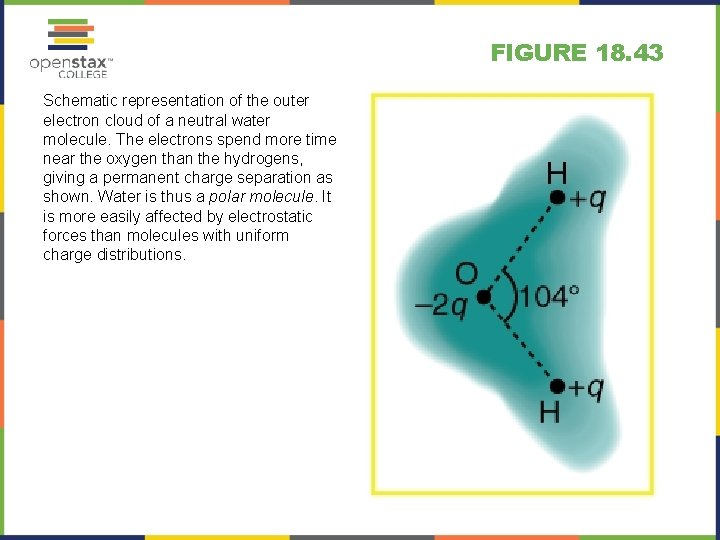FIGURE 18. 43 Schematic representation of the outer electron cloud of a neutral water molecule. The electrons spend more time near the oxygen than the hydrogens, giving a permanent charge separation as shown. Water is thus a polar molecule. It is more easily affected by electrostatic forces than molecules with uniform charge distributions.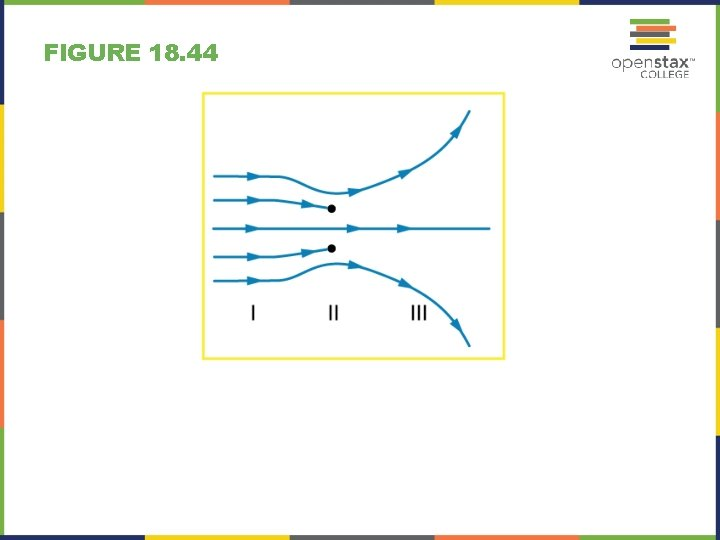FIGURE 18. 44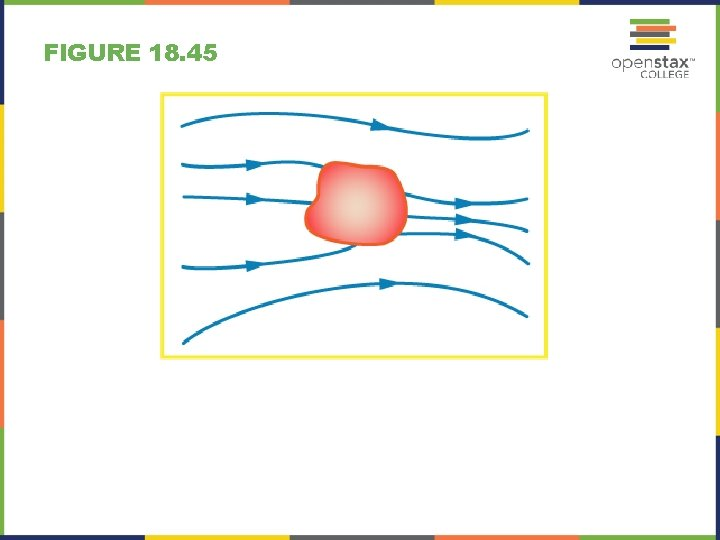FIGURE 18. 45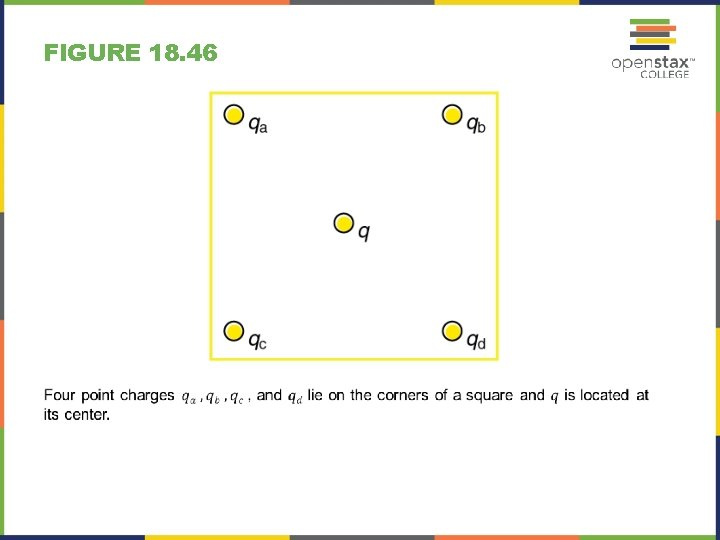FIGURE 18. 46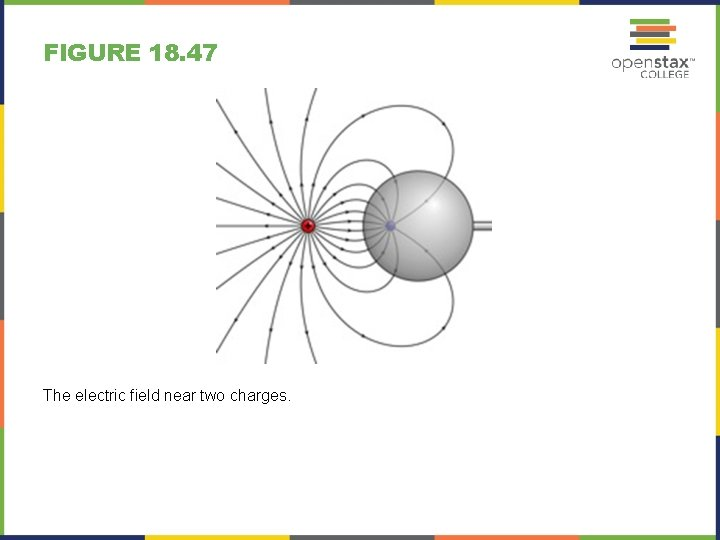FIGURE 18. 47 The electric field near two charges.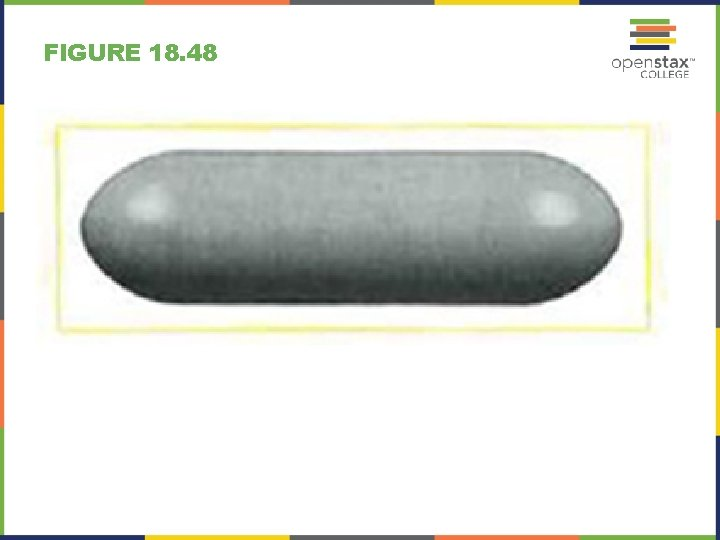FIGURE 18. 48FIGURE 18. 49FIGURE 18. 50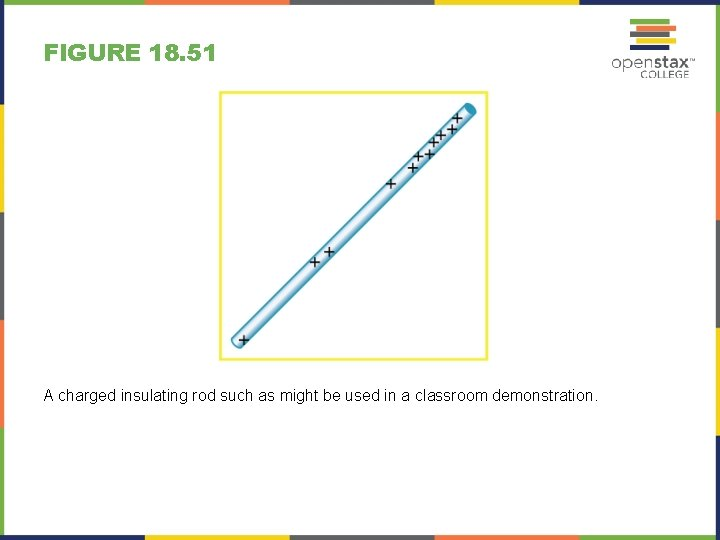FIGURE 18. 51 A charged insulating rod such as might be used in a classroom demonstration.FIGURE 18. 52 (a) Point charges located at 3. 00, 8. 00, and 11. 0 cm along the x-axis. (b) Point charges located at 1. 00, 5. 00, 8. 00, and 14. 0 cm along the x-axis.FIGURE 18. 53FIGURE 18. 54 Point charges located at the corners of an equilateral triangle 25. 0 cm on a side.FIGURE 18. 55 Parallel conducting plates with opposite charges on them create a relatively uniform electric field used to accelerate electrons to the right. Those that go through the hole can be used to make a TV or computer screen glow or to produce X rays.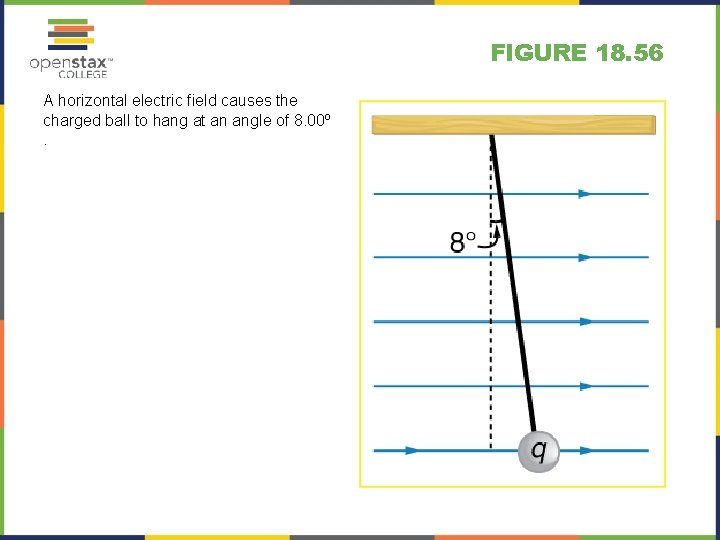FIGURE 18. 56 A horizontal electric field causes the charged ball to hang at an angle of 8. 00º .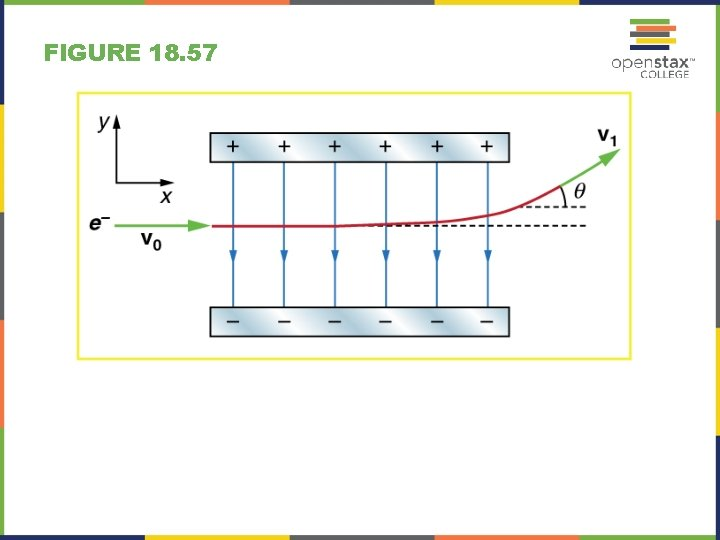FIGURE 18. 57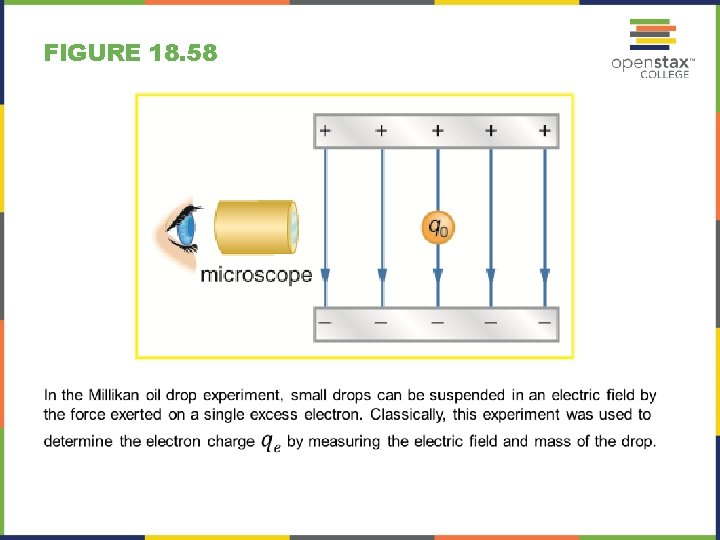FIGURE 18. 58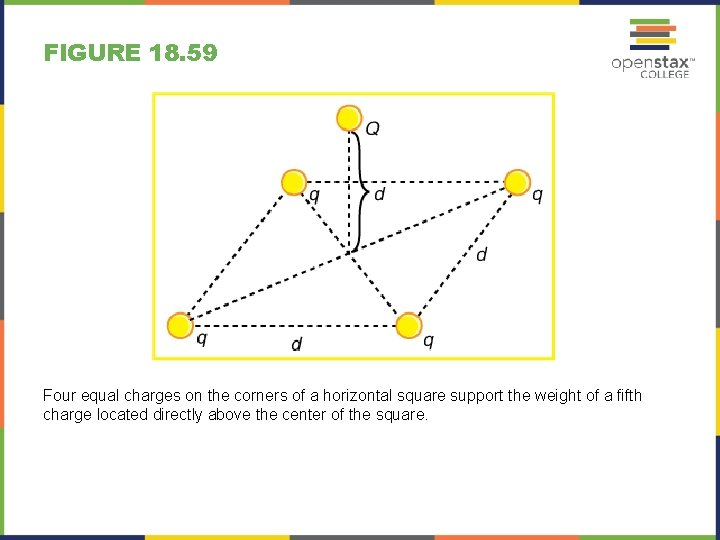FIGURE 18. 59 Four equal charges on the corners of a horizontal square support the weight of a fifth charge located directly above the center of the square.